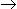# Chemical Engineering - Chemical Reaction Engineering

Exercise : Chemical Reaction Engineering - Section 10
1.
The conversion for a first order liquid phase reaction. AB in a CSTR is 50%. If another CSTR of the same volume is connected in series, then the % conversion at the exit of the second reactor will be
60
75
90
100
Explanation:
No answer description is available. Let's discuss.

2.
What is the dispersion number for a CSTR?
0
1
< 1
Explanation:
No answer description is available. Let's discuss.

3.
Rate of a chemical reaction is independent of the concentration of the reactants for a __________ reaction.
zero order
third order
consecutive
none of these
Explanation:
No answer description is available. Let's discuss.

4.
With increase in temperature, the rate constant obeying Arhenious equation
increases.
decreases.
decreases exponentially.
can either increase or decrease ; depends on the frequency factor.